Test: Chemical Kinetics - 1

# Test: Chemical Kinetics - 1

Test Description

## 20 Questions MCQ Test Physical Chemistry | Test: Chemical Kinetics - 1

Test: Chemical Kinetics - 1 for Chemistry 2022 is part of Physical Chemistry preparation. The Test: Chemical Kinetics - 1 questions and answers have been prepared according to the Chemistry exam syllabus.The Test: Chemical Kinetics - 1 MCQs are made for Chemistry 2022 Exam. Find important definitions, questions, notes, meanings, examples, exercises, MCQs and online tests for Test: Chemical Kinetics - 1 below.
Solutions of Test: Chemical Kinetics - 1 questions in English are available as part of our Physical Chemistry for Chemistry & Test: Chemical Kinetics - 1 solutions in Hindi for Physical Chemistry course. Download more important topics, notes, lectures and mock test series for Chemistry Exam by signing up for free. Attempt Test: Chemical Kinetics - 1 | 20 questions in 60 minutes | Mock test for Chemistry preparation | Free important questions MCQ to study Physical Chemistry for Chemistry Exam | Download free PDF with solutions
 1 Crore+ students have signed up on EduRev. Have you?
Test: Chemical Kinetics - 1 - Question 1

### For which of the following remarks the concentration of a reactant decreases linearly with time. What is the order of the reaction?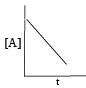Detailed Solution for Test: Chemical Kinetics - 1 - Question 1

For  Zero order reaction concentration of a reactant decreases linearly with time.

The integral form of zero order reactions can be rewritten as

[A]=–kt+[A0]

Comparing this equation with that of a straight line (y = mx + c), an [A] against t graph can be plotted to get a straight line with slope equal to ‘-k’ and intercept equal to [A]0 as shown below.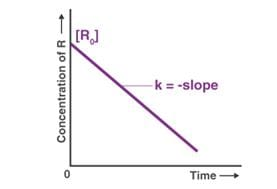Test: Chemical Kinetics - 1 - Question 2

### Consider the unimolecular reaction: A(g) → products, were made: (I) The reaction is second order at low pressure and become first order at high pressure. (II) The reaction is first order at low pressure and become second order at high pressure. (III) The reaction is zero order.Which of the fo llowing is correct?

Test: Chemical Kinetics - 1 - Question 3

### Examine the following first order consecutive reactions. The rate constant (in s-1 units) for each step. Is given above the arrow mark: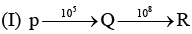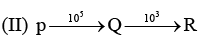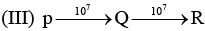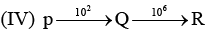Steady–state approximation can be applied to.

Detailed Solution for Test: Chemical Kinetics - 1 - Question 3

Steady state approximation can be applied to an intermediate that have a transient existence I.e. if the rate of deformation is greater than their rate of formation.

Test: Chemical Kinetics - 1 - Question 4

In the Lineweaver–Burk plot of (initial rate)–1 vs. (initial substrate concentration) –1 for an enzyme catalyzed reaction following Michaelis-Menten mechanism, the y–intercept is 5000 M–1 s. If the initial enzyme concentration is 1*10-9M, then turnover number is:

Test: Chemical Kinetics - 1 - Question 5

For the first order reaction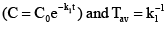After two average lives, concentration of the reactant is reduced to:

Detailed Solution for Test: Chemical Kinetics - 1 - Question 5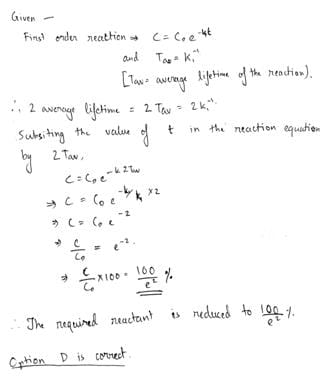Test: Chemical Kinetics - 1 - Question 6

Vmax and Km for an enzyme catalyzed reaction are 2.0 * 10-3 Ms -1 and 1.0 * 10-6 M, respectively. The rate of the reaction when the substrate concentration is 1.0 * 10-6 M  is

Test: Chemical Kinetics - 1 - Question 7

Experimentally determined rate law for the chemical reaction,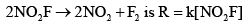The rate determining step consistent with the rate law is:

Test: Chemical Kinetics - 1 - Question 8

The rate of a chemical reaction doubles for every 10°C rise of temperature. If the temperature is raised by 50°C, the rate of the reaction increases by about

Detailed Solution for Test: Chemical Kinetics - 1 - Question 8

The rate of a chemical reaction doubles for every 10oC rise of temperature. If the temperature is raised by 50oC, the rate of the reaction increases by about 32 times.
50/10 = 5
2= 32

Test: Chemical Kinetics - 1 - Question 9

For an enzyme-substrate reaction, a plot between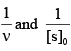yields a slope of 40 s. If the enzyme concentration is 2.5 μM , then the catalytic efficiency of the enzyme is:

Test: Chemical Kinetics - 1 - Question 10

Acid hydrolysis of ester in first order reaction and rate constant is given by,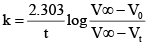Where, V0, Vt and V , are the volume of standard NaOH required to neutralize acid present as a given time, if ester is 50% neutralized then

Test: Chemical Kinetics - 1 - Question 11

For the reaction, A (g) + 2B(g) → C(g ) + D (g) ,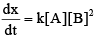Initial pressure of A and B are respectively 0.60 atm and 0.80 atm. At a time when pressure of C is 0.20 atm, rate of the reaction, relative to the initial value is:

Test: Chemical Kinetics - 1 - Question 12

If 60% of a first order reaction was completed in 60 minutes, 50% of the same reaction would be completed in approximately

Test: Chemical Kinetics - 1 - Question 13

The decomposition of ozone to oxygen,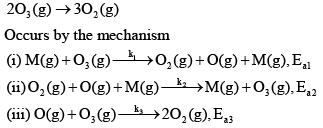Where, M is the catalyst molecule.
K’ are rate constants and Ea1 is the act ivat ion energy for the elementary step.

Q.

Under the steady state approximation for the intermediates, the rate of decomposition of ozone,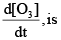Detailed Solution for Test: Chemical Kinetics - 1 - Question 13

Decomposition of ozone 2O3 --------> 3O2
The reaction is known to occur by the following mechanism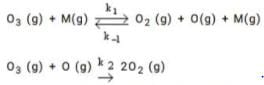Here M is the molecule that collides with O3 molecule and decompose the latter through a collision. The intermediate in this reaction is O which we treat using the steady state approximation.
The rate equation for [O] is d[O]/dt = k1[O3][M] - k-1[O2][O][M] - k2[O3][O]
Setting d[O]/dt = 0, we get the concentration of [O] as
[O] = k1[O3][M]/{k-1[O2][M] + k2[O3]}
The rate of change of O3 is given by
d[O3]/dt = -k1[O3][M] + k-1[O2][O][M] - k-2[O][O3]
Using SSA for [O] we have,
d[O3]/dt = 2k1k2[O3]2[M]/{k-1[O2][M] + k2[O3]}

Test: Chemical Kinetics - 1 - Question 14

The decomposition of ozone to oxygen,Where, M is the catalyst molecule.
K’ are rate constants and Ea1 is the act ivat ion energy for the elementary step.

Q.

Assuming k3[O3]>>k2[O2][M], the activation energy of the overall reaction is:

Detailed Solution for Test: Chemical Kinetics - 1 - Question 14

ATQ: B gives the resultant reaction in terms of O2, O3 and O only.

Test: Chemical Kinetics - 1 - Question 15

For the reaction shown below,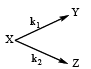The value of k1 is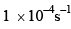. If the reaction starts from X, the ratio of the concentration of Y and Z at any given time during the course of the reaction is found to be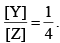The value of k2 is

Test: Chemical Kinetics - 1 - Question 16

At T = 300K, the thermal energy [kbT] in cm–1 is approximately:

Test: Chemical Kinetics - 1 - Question 17

For the reaction,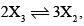the rate of formation of X2 is:

Test: Chemical Kinetics - 1 - Question 18

In a homogenous catalytic reaction, 1.0 M of a substrate band 1.0 μM of a catalyst yields 1.0 mM of a product in 10 s. The turnover frequency (TOF) of the reaction (s-1) is.

Test: Chemical Kinetics - 1 - Question 19

The value of the rate constant for the gas phase reaction, 2NO2 +F2 →2NO2F is 38 dm3 mol–1s–1 at 300K. The order of the reaction is:

Test: Chemical Kinetics - 1 - Question 20

A reaction follows second order rate law,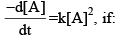Detailed Solution for Test: Chemical Kinetics - 1 - Question 20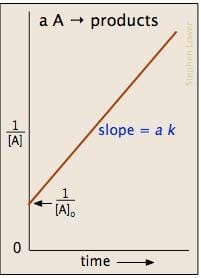## Physical Chemistry

83 videos|106 docs|31 tests
 Use Code STAYHOME200 and get INR 200 additional OFF Use Coupon Code
Information about Test: Chemical Kinetics - 1 Page
In this test you can find the Exam questions for Test: Chemical Kinetics - 1 solved & explained in the simplest way possible. Besides giving Questions and answers for Test: Chemical Kinetics - 1, EduRev gives you an ample number of Online tests for practice

## Physical Chemistry

83 videos|106 docs|31 tests